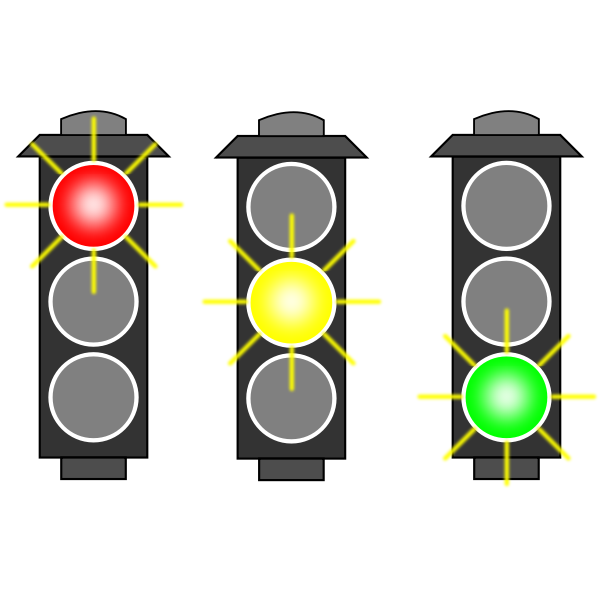PyCSP3
CSP  easy

# Problem Traffic Lights

From CSPLib (Problem 16): Consider a four way traffic junction with eight traffic lights. Four of the traffic lights are for the vehicles and can be represented by the variables $v_0$ to $v_3$ with domains {r,ry,g,y} (for red, red-yellow, green and yellow). The other four traffic lights are for the pedestrians and can be represented by the variables $p_0$ to $p_3$ with domains {r,g}. The constraints on these variables can be modeled by quaternary constraints on $(v_i, p_i, v_j, p_j)$ for $0 \leq i < 4, j=(1+i)\, \mathtt{mod}\, 4$ which allow just the tuples {(r,r,g,g), (ry,r,y,r), (g,g,r,r), (y,r,ry,r)}.’’

How to adjust traffic lights? Image from freesvg.orgTo build a CSP (Constraint Satisfaction Problem) model, we need first to import the library PyCSP$^3$:

from pycsp3 import *


Then, we need some data. Actually, we just need a few constants.

R, RY, G, Y = "red", "red-yellow", "green", "yellow"


We start our CSP model by introducing an array $v$ of 4 variables, one per vehicle traffic light.

# v[i] is the color for the ith vehicle traffic light
v = VarArray(size=4, dom={R, RY, G, Y})


We introduce a second array $p$ of 4 variables, one per pedestrian traffic light.

# p[i] is the color for the ith pedestrian traffic light
p = VarArray(size=4, dom={R, G})


We can display the structure of the arrays, as well as the domain of the first variable (note that all variables have the same domain).

print("Array v: ", v)
print("Array p: ", p)
print("Domain of any variable: ", v.dom)

Array v:  [v, v, v, v]
Array p:  [p, p, p, p]
Domain of any variable:  green red red-yellow yellow


As indicated in the statement of the problem, we need a table specifying the right combinations of values.

table = {(R, R, G, G), (RY, R, Y, R), (G, G, R, R), (Y, R, RY, R)}


We can then post 4 quaternary constraints Extension by using the table.

satisfy(
(v[i], p[i], v[(i + 1) % 4], p[(i + 1) % 4]) in table for i in range(4)
);


We can display the internal representation of the two posted constraints; this way, although a little bit technical, we can see that the arguments of teh four constraints are correct.

print(posted())

extension(list:[v, p, v, p], supports:(green,green,red,red)(red,red,green,green)(red-yellow,red,yellow,red)(yellow,red,red-yellow,red))
extension(list:[v, p, v, p], supports:(green,green,red,red)(red,red,green,green)(red-yellow,red,yellow,red)(yellow,red,red-yellow,red))
extension(list:[v, p, v, p], supports:(green,green,red,red)(red,red,green,green)(red-yellow,red,yellow,red)(yellow,red,red-yellow,red))
extension(list:[v, p, v, p], supports:(green,green,red,red)(red,red,green,green)(red-yellow,red,yellow,red)(yellow,red,red-yellow,red))


Interestingly, by calling the function solve(), we can check that the problem is satisfiable (SAT). We can also display the found solution. Here, we call the function values() that collects the values assigned to a specified list of variables.

if solve() is SAT:
print("Colors for vehicles  : ", values(v))
print("Colors fo pedestrians: ", values(p))


Colors for vehicles  :  ['green', 'red', 'green', 'red']
Colors fo pedestrians:  ['green', 'red', 'green', 'red']


We can enumerate the solutions as follows:

if solve(sols=10) is SAT:
for i in range(n_solutions()):
print(f"Solution {i+1}: {values(v, sol=i)} {values(p, sol=i)}")

Solution 1: ['green', 'red', 'green', 'red'] ['green', 'red', 'green', 'red']
Solution 2: ['red-yellow', 'yellow', 'red-yellow', 'yellow'] ['red', 'red', 'red', 'red']
Solution 3: ['yellow', 'red-yellow', 'yellow', 'red-yellow'] ['red', 'red', 'red', 'red']
Solution 4: ['red', 'green', 'red', 'green'] ['red', 'green', 'red', 'green']